# 1 Load requiered R packages

``library(NestLink)``

# 2 Display ESP

ESP_Prediction was generated using an application https://genepattern.broadinstitute.org (???).

``````library(ggplot2)
ESP <- rbind(getFC(), getNB())``````
``## snapshotDate(): 2020-10-02``
``## see ?NestLink and browseVignettes('NestLink') for documentation``
``## loading from cache``
``## snapshotDate(): 2020-10-02``
``## see ?NestLink and browseVignettes('NestLink') for documentation``
``## loading from cache``
``````p <- ggplot(ESP, aes(x = ESP_Prediction, fill = cond)) +
geom_histogram(bins = 50, alpha = 0.4, position="identity") +
labs(x = "detectability in LC-MS (ESP prediction)") +
theme(panel.grid.major = element_blank(), panel.grid.minor = element_blank(),
panel.background = element_blank(), axis.line = element_line(colour = "black"))
print(p)``````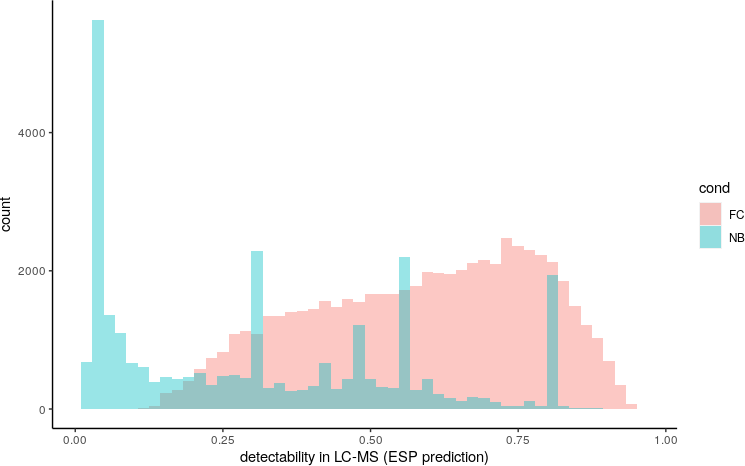``ESP <- rbind(getFC(), NB.unambiguous(getNB()))``
``## snapshotDate(): 2020-10-02``
``## see ?NestLink and browseVignettes('NestLink') for documentation``
``## loading from cache``
``## snapshotDate(): 2020-10-02``
``## see ?NestLink and browseVignettes('NestLink') for documentation``
``## loading from cache``
``````p <- ggplot(ESP, aes(x = ESP_Prediction, fill = cond)) +
geom_histogram(bins = 50, alpha = 0.4, position="identity") +
labs(x = "detectability in LC-MS (ESP prediction)") +
theme(panel.grid.major = element_blank(), panel.grid.minor = element_blank(),
panel.background = element_blank(), axis.line = element_line(colour = "black"))
print(p)``````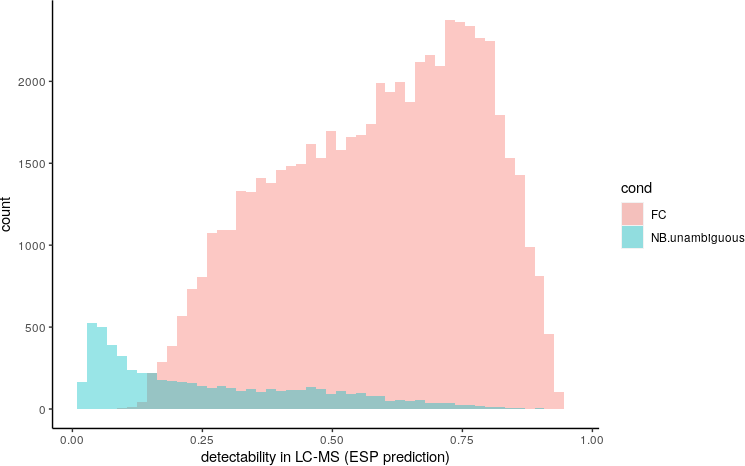``ESP <- rbind(getFC(), NB.unique(getNB()))``
``## snapshotDate(): 2020-10-02``
``## see ?NestLink and browseVignettes('NestLink') for documentation``
``## loading from cache``
``## snapshotDate(): 2020-10-02``
``## see ?NestLink and browseVignettes('NestLink') for documentation``
``## loading from cache``
``````p <- ggplot(ESP, aes(x = ESP_Prediction, fill = cond)) +
geom_histogram(bins = 50, alpha = 0.4, position="identity") +
labs(x = "detectability in LC-MS (ESP prediction)") +
theme(panel.grid.major = element_blank(), panel.grid.minor = element_blank(),
panel.background = element_blank(), axis.line = element_line(colour = "black"))
print(p)``````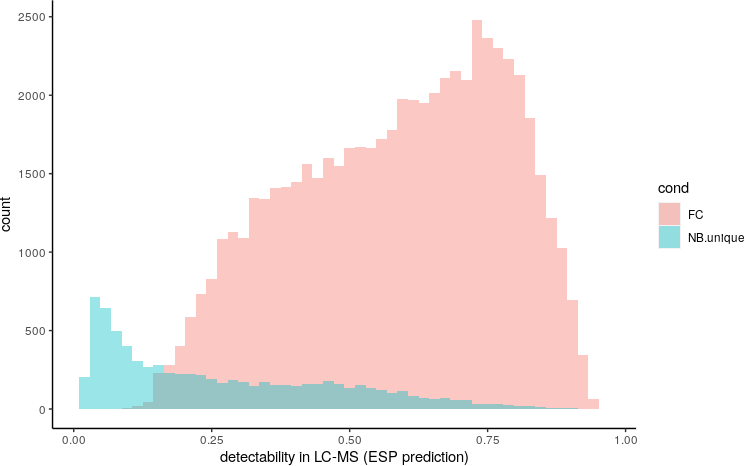``ESP <- rbind(getNB(), NB.unambiguous(getNB()), NB.unique(getNB()))``
``## snapshotDate(): 2020-10-02``
``## see ?NestLink and browseVignettes('NestLink') for documentation``
``## loading from cache``
``## snapshotDate(): 2020-10-02``
``## see ?NestLink and browseVignettes('NestLink') for documentation``
``## loading from cache``
``## snapshotDate(): 2020-10-02``
``## see ?NestLink and browseVignettes('NestLink') for documentation``
``## loading from cache``
``````p <- ggplot(ESP, aes(x = ESP_Prediction, fill = cond)) +
geom_histogram(bins = 50, alpha = 0.4, position="identity") +
labs(x = "detectability in LC-MS (ESP prediction)") +
theme(panel.grid.major = element_blank(), panel.grid.minor = element_blank(),
panel.background = element_blank(), axis.line = element_line(colour = "black"))
print(p)``````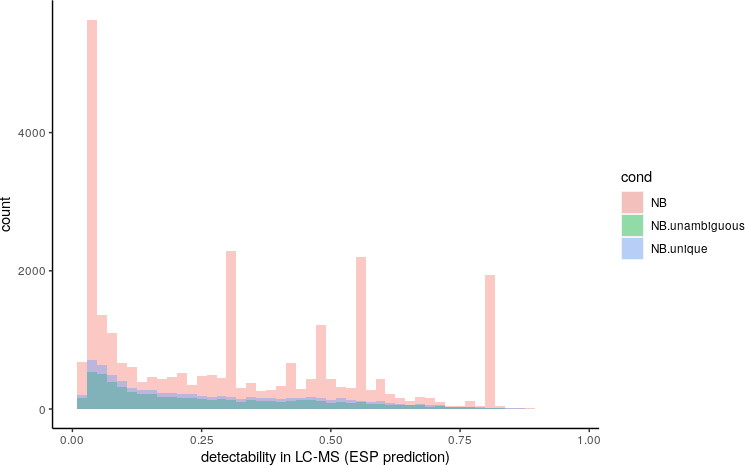``table(ESP\$cond)``
``````##
##             NB NB.unambiguous      NB.unique
##          27681           5832           7705``````

# 3 Charge State Frequency

## 3.1 Load workunit 160118

``````# library(ExperimentHub)
# eh <- ExperimentHub();

WU <- WU160118``````

filtering

``````PATTERN <- "^GS[ASTNQDEFVLYWGP]{7}(WR|WLTVR|WQEGGR|WLR|WQSR)\$"

idx <- grepl(PATTERN, WU\$pep_seq)
WU <- WU[idx & WU\$pep_score > 25,]``````

determine charge state frequency through counting

``````WU\$chargeInt <- as.integer(substr(WU\$query_charge, 0, 1))
table(WU\$chargeInt)``````
``````##
##     2     3     4
## 33392   157     1``````

in percent

``round(100 * (table(WU\$chargeInt) / nrow(WU)), 1)``
``````##
##    2    3    4
## 99.5  0.5  0.0``````

as histograms

``````library(scales)
ggplot(WU, aes(x = moverz * chargeInt, fill = (query_charge),
colour = (query_charge))) +
facet_wrap(~ datfilename, ncol = 2) +
geom_histogram(binwidth= 10, alpha=.3, position="identity") +
labs(title = "Precursor mass to charge frequency plot",
subtitle = "Plotting frequency of precursor masses for each charge state",
x = "Precursor mass [neutral mass]",
y = "Frequency [counts]",
fill = "Charge State",
colour = "Charge State") +
scale_x_continuous(breaks = pretty_breaks(8)) +
theme_light()``````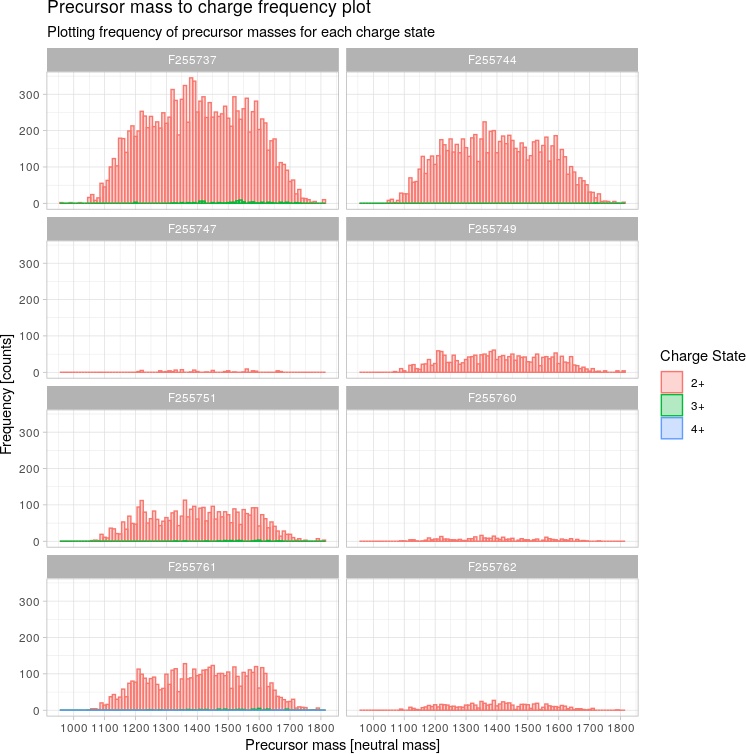We confirmed this prediction by experimental data and found that 99.9 percent of flycode precursor ions correspond to doubly charge species (data not shown). The omission of positively charged residues is also critical in order to render trypsin a site-specific protease.

## 3.2 FlyCode Mass Distribution

``````WU\$suffix <- substr(WU\$pep_seq, 10, 100)

ggplot(WU, aes(x = moverz * chargeInt, fill = suffix, colour = suffix)) +
geom_histogram(binwidth= 10, alpha=.2, position="identity") +
#facet_wrap(~ substr(pep_seq, 10, 100)) +
theme_light()``````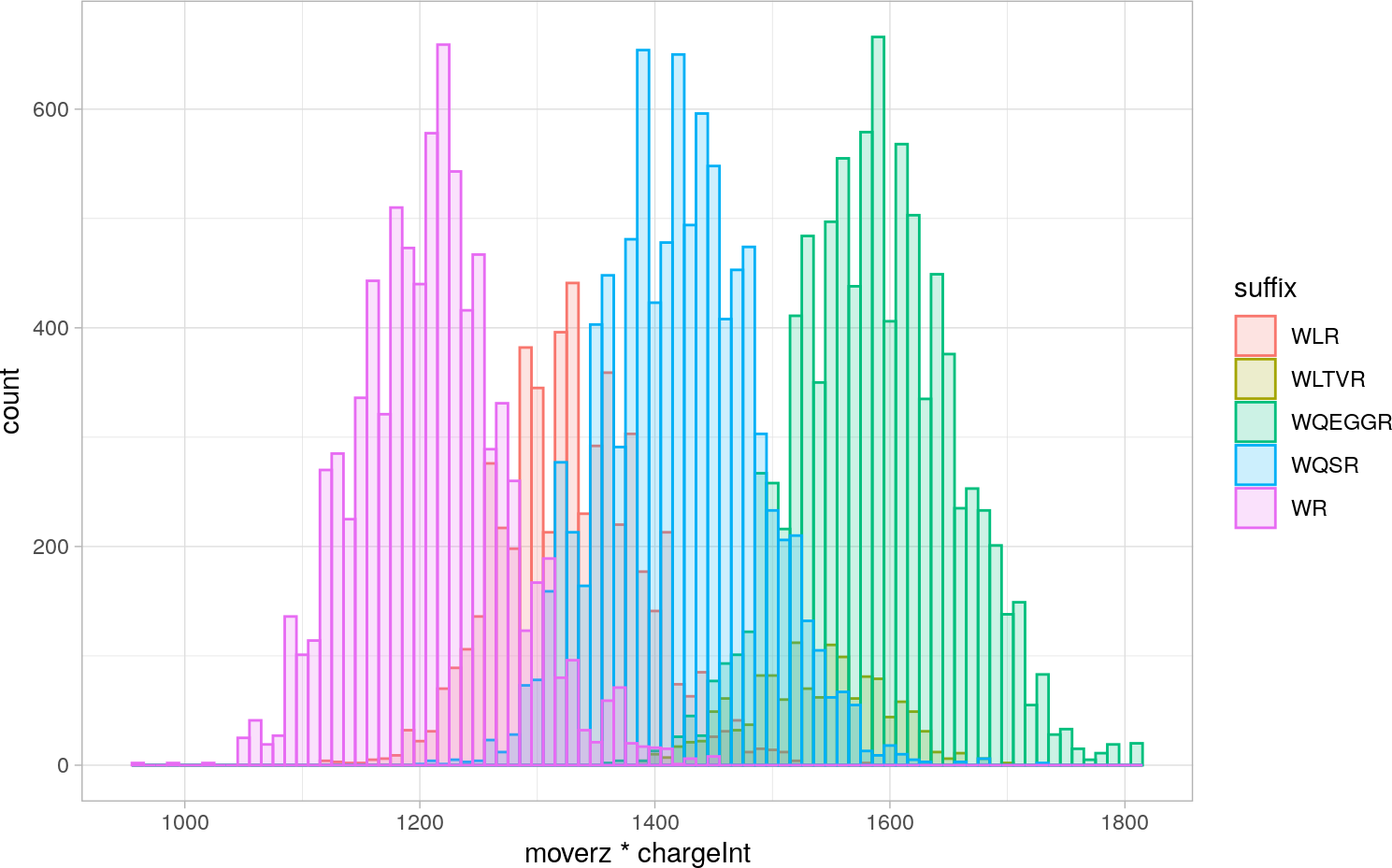``````ggplot(WU, aes(x = moverz * chargeInt, fill = suffix)) +
geom_histogram(binwidth= 10, alpha=.9, position="identity") +
facet_wrap(~ substr(pep_seq, 10, 100)) +
theme_light()``````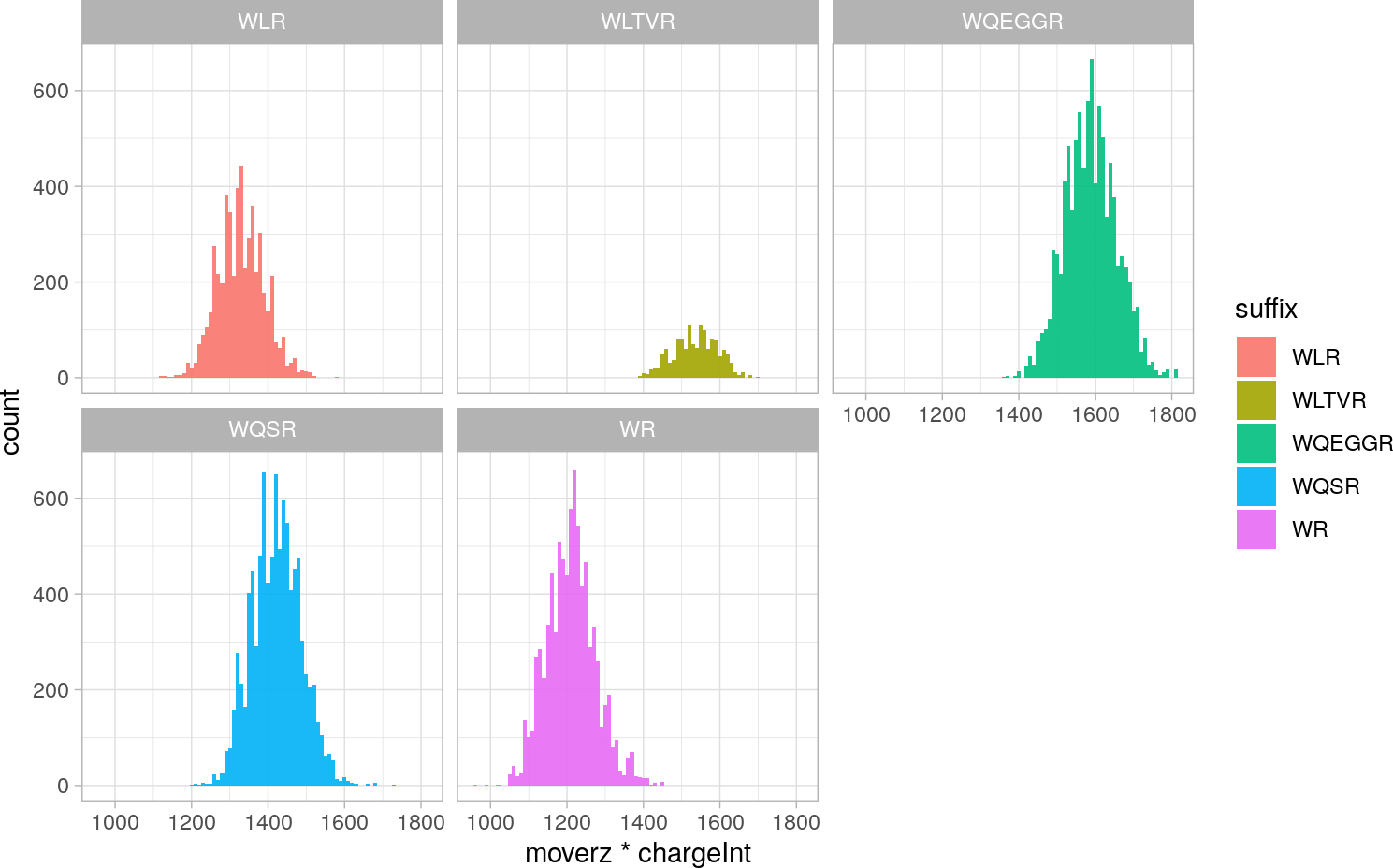## 3.3 Mascot Ion Score Distribution

``````ggplot(WU, aes(x = pep_score, fill = query_charge, colour = query_charge)) +
geom_histogram(binwidth= 2, alpha=.5, position="identity") +
facet_wrap(~ substr(pep_seq, 10, 100)) +
theme_light()``````